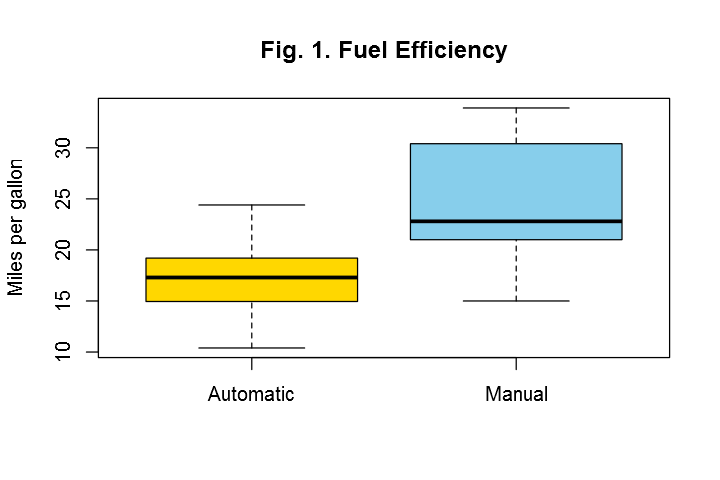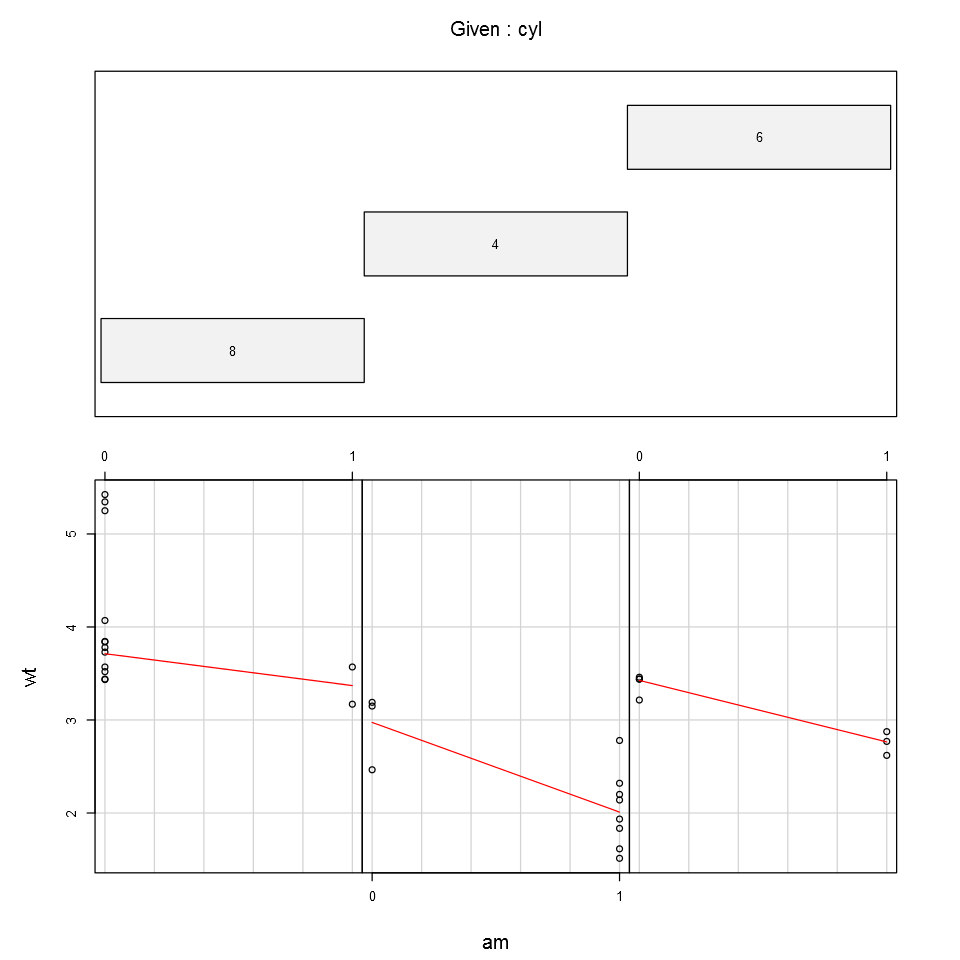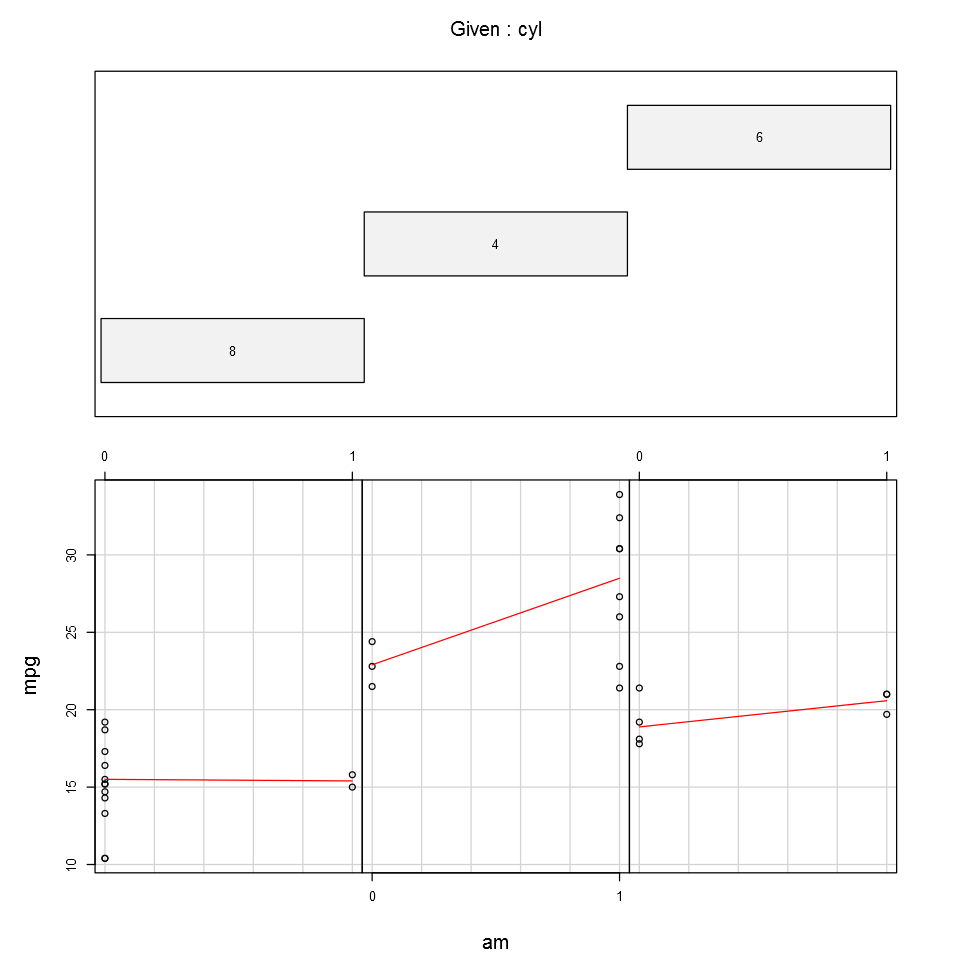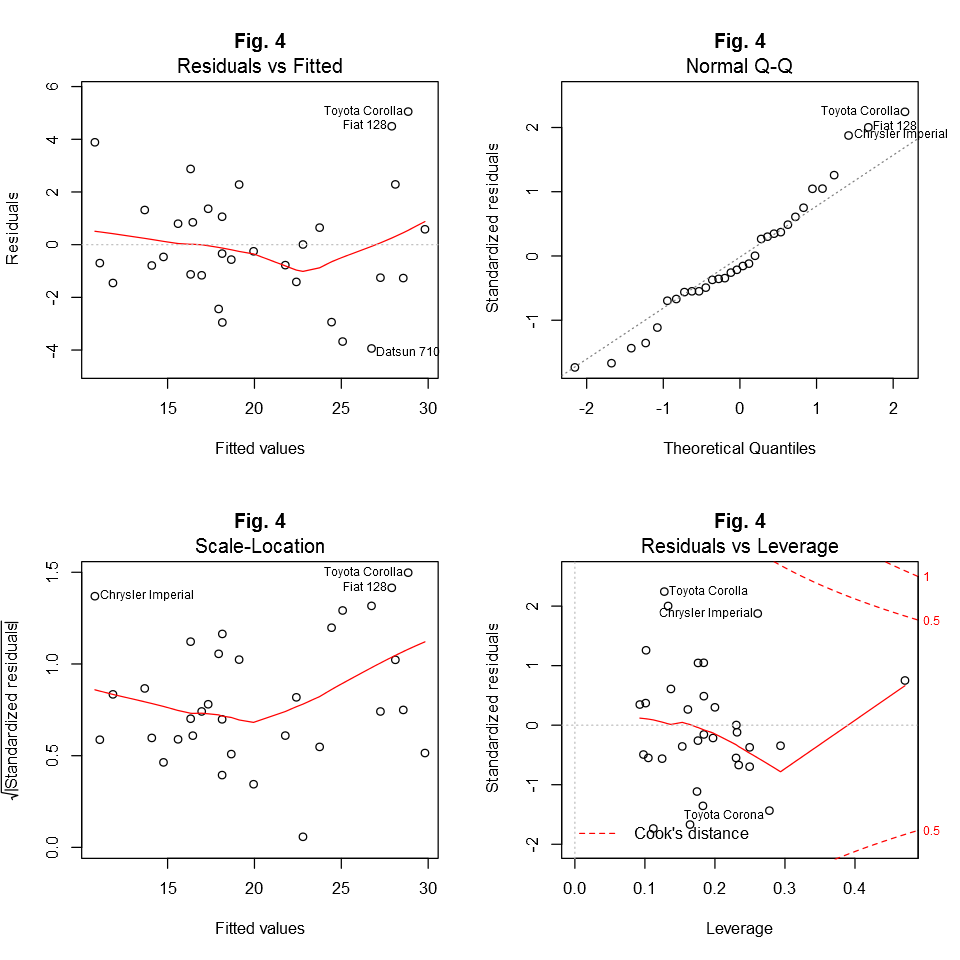# Fisseha Berhane, PhD

#### Data Scientist443-970-2353[email protected] CV Resume## Car Fuel Efficiency and Transmission Type¶

### Summary¶

In this analysis, the relationship between a set of variables and miles per gallon (MPG) is explored using the mtcars dataset. Particularly, the MPG difference between automatic and manual transmissions is evaluated and quantified. The final selected multivariable linear r egression model shows that manual transmission cars have about 1.81 miles more per gallon as compared to automatic transmission cars, keeping other variables constant. However, caution should be practiced in interpreting this assocation: (1) the dataset is relatively old and may not reflect present conditions, (2) the sample size is small as compared to the number of features and conclusions derived from models employing small sample size could be biased, (3) the association between transmission type and MPG is particularly clear with four cylinder cars which are light weight as compared to six and eight cylinder cars. (4) Automatic transmission cars weight more than manual transmission cars. Therefore, the difference in weight could be the main reason for the association found between MPG and transmission type.

## Exploratory Data Analysis¶

In :
data(mtcars)  #loading dataset
str(mtcars)   # understand the covariates
names(mtcars)

'data.frame':	32 obs. of  11 variables:
$mpg : num 21 21 22.8 21.4 18.7 18.1 14.3 24.4 22.8 19.2 ...$ cyl : num  6 6 4 6 8 6 8 4 4 6 ...
$disp: num 160 160 108 258 360 ...$ hp  : num  110 110 93 110 175 105 245 62 95 123 ...
$drat: num 3.9 3.9 3.85 3.08 3.15 2.76 3.21 3.69 3.92 3.92 ...$ wt  : num  2.62 2.88 2.32 3.21 3.44 ...
$qsec: num 16.5 17 18.6 19.4 17 ...$ vs  : num  0 0 1 1 0 1 0 1 1 1 ...
$am : num 1 1 1 0 0 0 0 0 0 0 ...$ gear: num  4 4 4 3 3 3 3 4 4 4 ...
$carb: num 4 4 1 1 2 1 4 2 2 4 ...  Out: 1. "mpg" 2. "cyl" 3. "disp" 4. "hp" 5. "drat" 6. "wt" 7. "qsec" 8. "vs" 9. "am" 10. "gear" 11. "carb" From the above set of commands and using help(mtcars), I have noticed that all variables are loaded as numeric even if some of them are factor viarables. Before, starting our analysis, let’s first change the factor vaiables and make the most frequent event as a reference. In : mtcars$cyl<-relevel(as.factor(mtcars$cyl), ref='8') mtcars$vs<-relevel(as.factor(mtcars$vs), ref='0') mtcars$am<-relevel(as.factor(mtcars$am), ref='0',labels=c('Automatic','Manual')) mtcars$gear<-relevel(as.factor(mtcars$gear), ref='3') mtcars$carb<-relevel(as.factor(mtcars$carb), ref='2')  Using pairs(mtcars), we get that fuel consumption increases as the number of cylinders (cyl), displacement (disp), horsepower (hp), and weight (wt) increase. That is to say, as these variables increase, the Miles per gallon (MPG) of cars dereases. We also observe that in this old dataset, manual tranmsion cars (am=1) have higher MPG than automatic transmission cars (am=0) as can be observed in Fig. 1. Let’s use t test and see if the difference in the mean MPG of automatic and maual transmisssion cars is significantly different from zero. In : t.test(mtcars$mpg[mtcars$am==0],mtcars$mp[mtcars$am==1])  Out:  Welch Two Sample t-test data: mtcars$mpg[mtcars$am == 0] and mtcars$mp[mtcars$am == 1] t = -3.7671, df = 18.332, p-value = 0.001374 alternative hypothesis: true difference in means is not equal to 0 95 percent confidence interval: -11.280194 -3.209684 sample estimates: mean of x mean of y 17.14737 24.39231  We see from the test results that the p-value is very small and hence we reject the null hypothesis and accept the alternative hypothesis that the true mean difference of MPG of cars with automatic and manual transmissions is not zero. We see from this old dataset that manual transmission cars are more fuel-efficient with more MPG as compared to automatic transmission cars. However, we also see from Fig. 2 that manual transmission cars weigh less as compared to automatic transmission cars. Hence, weight could be the cause for the observed difference in MPG as heavy cars use more power. From the conditional plot shown in the appendix (Fig. 3), one cay say that clear difference in MPG between automatic and manual transmissions is observed with in those that have four cylinders. Neverthless, it is important to note that we have very small sample size of cars with six and eight cylinders that have manual transmission and this may bias our conclusions. ### Multivariate Linear Regression Models¶ Now, let’s fit a multivariate regression model taking MPG as outcome (response). First, let’s consider all features and see the model’s performance. In : modelA<-lm(mpg~., data=mtcars)  In modelA all features are not significant. Moreover, from the adjusted R-square, the model has included variables that are not important. Now, let’s use atomatic model selection using all covariates and the step function which finds a good compromise of model simplicity and R-square. The step function is formalized by the Akaike information criterion (AIC). In : modelB<-step(modelA,direction ="both")  Now, let’s see the variables selected by the step function. In : modelB$coefficients

Out:
(Intercept)
31.5446485781016
cyl4
2.16367532317874
cyl6
-0.867669167324412
hp
-0.0321094299908177
wt
-2.496829420354
am1
1.80921138294121

So from modelB, we see that the step function selected variables wt (weight), hp (horse power), cyl (number of cylinders) and am (transmission type) as features. From the coeffiencets, we see that manual transmission cars have 1.81 miles more per gallon as compared to automatic transmission cars, keeping other factors constant. Moreover, the model says that MPG deacrease by about 2.5 Miles as weight increases by 1000lb, keeping other factors constant. On the other hand, we see that four cylinder cars have about 2.2 more MPG than eight cylinder cars, keeping other variables constant.

### Model Diagnostics¶

From Fig. 4, we see that the residuals vs fitted plot is not completely random. Further, the normal Q-Q plot has points that deviate from the normal line. These points suggest that the model is far from perfect.

### Conclusions¶

In this analysis, it is found that manual transmission cars have 1.81 milles per gallon more as compared to automatic transmission cars. However, we have to note that the datset is old and it may not be representative of the current conditions. Further, as shown in Fig.3., the association between transmission and MPG is clear for four cylinder cars. The other important point worth mentioning is the fact that the data has small sample size and deriving strong conclusions based on the dataset may not be warranted. Last but not least, manual transmission cars weigh less than automatic transmission cars (Fig. 2) and this could be the reaseon for the observed association between MPG and transmission type.

### Appendix¶

In :
boxplot(mpg~am, data=mtcars, main ='Fig. 1. Fuel Efficiency',
ylab='Miles per gallon',names=c("Automatic","Manual"),notch=FALSE, col=(c("gold","skyblue")))In :
coplot(wt ~ am |cyl, data = mtcars,
panel = panel.smooth, rows = 1, main ="Fig. 2")In :
coplot(mpg ~ am | cyl, data = mtcars,    # cyl is the number of cylinders
panel = panel.smooth, rows = 1, main="Fig. 3")   # am is transmision type (0=automatic, 1=manual)In :
par(mfrow=c(2,2))
plot(modelB, main="Fig. 4")